# Identify which sets of quantum numbers are valid for an electron. Each set is ordered (n, l, ml, ms)

Identify which sets of quantum numbers are valid for an electron. Each set is ordered (n, l, ml, ms).

Concepts and reason
Four quantum numbers: (n, l,ml,ms)
Four quantum numbers are used to describe the most probable position of the electron of an atom in space.
The Pauli Exclusion Principle:
No two electrons can have the same set of four quantum numbers.
Hund’s Rule:
Electrons fill each orbital first in a subshell with one electron before entering half-filled orbitals.
Aufbau Principle:
Electrons will occupy the lowest energy level orbital first. This is used to determine the electronic configuration of an atom or ion.

Fundamentals
Four quantum numbers and their symbols: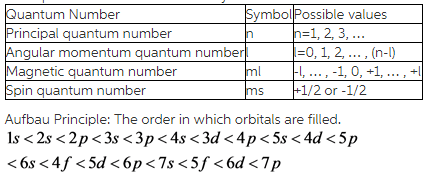(A)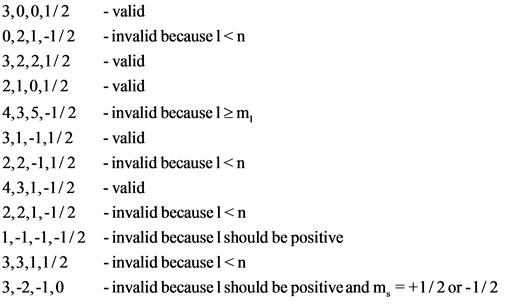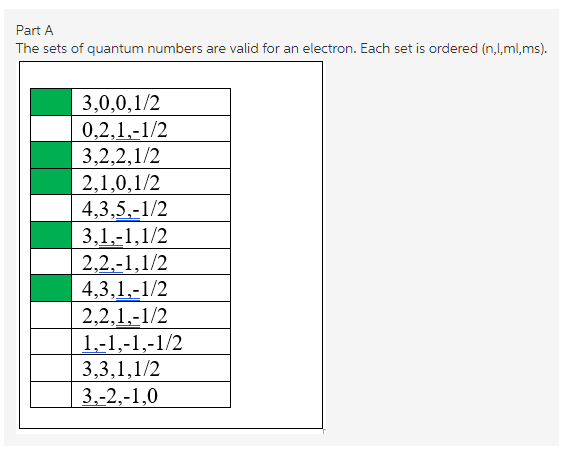Explanation:
The valid sets of quantum numbers are identified and reasons are mentioned for invalid sets.

(B)
Symbolic representation of beryllium element with atomic number and mass number:
Beryllium =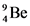It has totally four electrons.
Ground state electronic configuration of beryllium =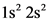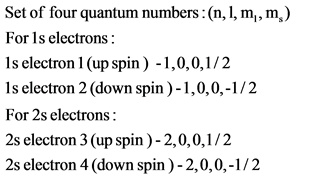Explanation:
A neutral beryllium is found in a Group 2 (alkaline earth metals) family. It has two electrons in its core shell and two more electrons in its valence shell. The electronic configuration of a neutral beryllium atom is written as. The s electrons differ only in spin quantum numbers because the s subshell has one orbital. The principal quantum of 1s orbital is n=1 and 2s orbital is n=2. Thus, the sets of quantum numbers for four electrons in the ground state of a neutral beryllium atom are (1,0,0,1/2),( 1,0,0,-1/2),( 2,0,0,1/2) and (2,0,0,-1/2).